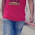### Challenging question

Question 1
Prove that $\frac{\tan\theta}{1-\cot\theta} + \frac{\cot\theta}{1-\tan\theta} = 1 + \sec\theta\csc\theta$

From LHS (Do not need to show this in answer, I am doing this to explain why we need to multiply by $\cos\theta - \sin\theta$ later on )
\begin{aligned} \frac{\sin\theta}{\cos\theta} \left(\frac{\sin\theta}{\sin\theta-\cos\theta}\right) + \frac{\cos\theta}{\sin\theta} \left(\frac{\cos\theta}{\cos\theta - \sin\theta} \right) &= \frac{-\sin^3 \theta + \cos^3 \theta}{\sin\theta \cos\theta (\cos\theta - \sin\theta)} \\ \end{aligned}

From RHS
\begin{aligned} 1 + \frac{1}{\sin\theta}\frac{1}{\cos\theta} &= \frac{\sin\theta \cos\theta + 1}{\sin\theta \cos\theta} \\ &= \frac{\sin\theta \cos\theta + 1}{\sin\theta \cos\theta} \left(\frac{\cos\theta - \sin\theta}{\cos\theta - \sin\theta} \right) \qquad \text{clue from LHS}\\ &= \frac{\sin\theta\cos^2\theta - \sin^2\theta\cos\theta + \cos\theta - \sin\theta}{\sin\theta \cos\theta (\cos\theta - \sin\theta)} \\ &= \frac{\sin\theta(1-\sin^2\theta) - (1-\cos^2\theta)\cos\theta + \cos\theta - \sin\theta}{\sin\theta \cos\theta (\cos\theta - \sin\theta)} \\ &= \frac{-\sin^3 \theta + \cos^3 \theta}{\sin\theta \cos\theta (\cos\theta - \sin\theta)} \\ &= \frac{\sin\theta}{\cos\theta} \left(\frac{\sin\theta}{\sin\theta-\cos\theta}\right) + \frac{\cos\theta}{\sin\theta} \left(\frac{\cos\theta}{\cos\theta - \sin\theta} \right) \\ &= \frac{\tan\theta}{1-\cot\theta} + \frac{\cot\theta}{1-\tan\theta} \end{aligned}

Note that the next question is not in the O level A Maths syllabus
Question 2
Differentiate with respect to x $\qquad y = \frac{x \sqrt{ x^2 + 1}}{(x + 1)^{\frac{2}{3}}}$

The simpler way is to use logarithmic differentiation
\begin{aligned} y &= \frac{x \sqrt{ x^2 + 1}}{(x + 1)^{\frac{2}{3}}} \\ \ln{y} &= \ln{x} + \frac{1}{2} \ln{(x^2 +1)} - \frac{2}{3} \ln{(x+1)} \\ \text{differentiate implicitly with respect to x } \\ \frac{1}{y} \frac{dy}{dx} &= \frac{1}{x} + \frac{1}{2} \frac{1}{x^2 + 1} 2x - \frac{2}{3}\frac{1}{x+1} \\ \end{aligned}

The other method is to use quotient rule
\begin{aligned} \frac{dy}{dx} &=\frac{(x+1)^\frac{2}{3} (x(\frac{1}{2})(x^2+1)^{-\frac{1}{2}}2x + \sqrt{ x^2 + 1}) -x \sqrt{ x^2 + 1}(\frac{2}{3}(x+1)^\frac{-1}{3}) }{(x + 1)^{\frac{4}{3}}} \end{aligned}

You would realize that it gets really messy if quotient rule is used. Hence implicit differentiation is recommended.

Note that the next question is not in the O level A Maths syllabus
Question 3
Find an equation of the curve that passes through the point (0, 1) and whose slope at (x, y) is 3xy

Given $\frac{dy}{dx} = 3xy$

\begin{aligned} \frac{dy}{dx} &= 3xy \\ \frac{1}{y} \frac{dy}{dx} &= 3x \\ \text{Integrate by seperating variables} \int \frac{1}{y} dy &= \int 3x dx \\ \ln{y} &= \frac{3x^2}{2} + c \\ \text{Substitute} x=0 \qquad y = 1 \\ c &= -\frac{3}{2} \\ \end{aligned}

1.plzz just make LHS =RHS

i mean just solve LHS so it is equal to RHS
thanks.
will vote u the best answer for sure
and in the 1st line u wrote the ques. wrong
u wrote 1-secQ but it is 1+secQ
although u have done it corrwct,....